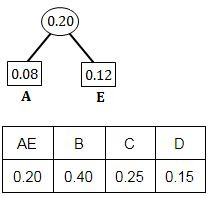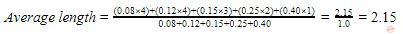Question 1

Given below are some algorithms, and some algorithm design paradigms

```A. Dijkstra’s Shortest Path                     1. Divide and Conquer
B. Floyd-Warshall algorithm to                  2. Dynamic Programming
compute all pairs shortest path
C. Binary search on a sorted array              3. Greedy design
D. Backtracking search on a graph               4. Depth-first search
```

Match the above algorithms on the left to the corresponding design paradigm they follow Codes:

 A 1-i, 2-iii, 3-i, 4-v. B 1-iii, 2-iii, 3-i, 4-v. C 1-iii, 2-ii, 3-i, 4-iv. D 1-iii, 2-ii, 3-i, 4-v.
Question 1 Explanation:
(1) Dijkstra’s Shortest Path using to find single source shortest path. It is purely based on Greedy technique because it always selects shortest path among all.
(2) → All Pair shortest path algorithm is using Dynamic Programming technique. It takes worst case time complexity is O(|V|3) and worst case space complexity is O(|V|2).
→ The Floyd–Warshall algorithm is an algorithm for finding shortest paths in a weighted graph with positive or negative edge weights (but with no negative cycles).
→ A single execution of the algorithm will find the lengths (summed weights) of the shortest paths between all pairs of vertices. Although it does not return details of the paths themselves, it is possible to reconstruct the paths with simple modifications to the algorithm.
(3) Binary search on a sorted array:
Search a sorted array by repeatedly dividing the search interval in half. Begin with an interval covering the whole array. If the value of the search key is less than the item in the middle of the interval, narrow the interval to the lower half. Otherwise narrow it to the upper half. Repeatedly check until the value is found or the interval is empty.
(4) Backtracking search on a graph uses Depth-first search
 Question 2
Which one of the following algorithm design techniques is used in finding all pairs of shortest distances in a graph?
 A Dynamic programming B Backtracking C Greedy D Divide and Conquer
Question 2 Explanation:
→ The Floyd-Warshall algorithm is an algorithm for finding shortest paths in a weighted graph with positive or negative edge weights (but with no negative cycles).
→ A single execution of the algorithm will find the lengths (summed weights) of shortest paths between all pairs of vertices
→ The Floyd-Warshall algorithm is an example of dynamic programming.
 Question 3

A text is made up of the characters A, B, C, D, E each occurring with the probability 0.08, 0.40, 0.25, 0.15 and 0.12 respectively. The optimal coding technique will have the average length of :

 A 2.4 B 1.87 C 3 D 2.15
Algorithms       Algorithm-Paradigms       UGC-NET CS 2018 JUNE Paper-2
Question 3 Explanation:Step 1: Select two characters with smallest probabilities and merge them.Step 2: Select two characters from Step 1 with smallest probabilities and merge them.Step 3: Select two characters (from Step 2) with smallest probabilities and merge them.Step 4: Merge the remaining two probabilities.A = 1000 (4-bits)
E = 1001 (4-bits)
D = 101 (3-bits)
C = 11 (2-bits)
B = 0 (1-bit)Question 4

Match the following with respect to algorithm paradigms :

```          List-I                        List-II
(a) The 8-Queens problem               (i) Dynamic programming
(b) Single-Source shortest paths      (ii) Divide and conquer
(c) STRASSEN’s Matrix multiplication (iii) Greedy approach
(d) Optimal binary search trees       (iv) Backtracking

```

 A (a)-(iv), (b)-(i), (c)-(iii), (d)-(ii) B (a)-(iv), (b)-(iii), (c)-(i), (d)-(ii) C (a)-(iii), (b)-(iv), (c)-(ii), (d)-(i) D (a)-(iv), (b)-(iii), (c)-(ii), (d)-(i)
Algorithms       Algorithm-Paradigms       UGC-NET CS 2018 JUNE Paper-2
Question 4 Explanation:
8-Queens problem use Backtracking.
Single-Source shortest paths use Greedy approach.
STRASSEN’s Matrix multiplication use Divide and conquer technique.
Optimal binary search trees use Dynamic programming.
 Question 5
What is the type of the algorithm used in solving the 4 Queens problem?
 A Greedy B Branch and bound C Dynamic Programming D Backtracking
Algorithms       Algorithm-Paradigms       Nielit Scientist-B IT 4-12-2016
Question 5 Explanation:
N-Queen problem:​ an arrangement of N queens on a chess board, such that no queen can attack any other queens on the board.The chess queens can attack in any direction as horizontal, vertical, horizontal and diagonal way. A binary matrix is used to display the positions of N Queens, where no queens can attack other queens.
Backtracking is a general algorithm for finding all (or some) solutions to some computational problems, notably constraint satisfaction problems, that incrementally builds candidates to the solutions, and abandons a candidate ("backtracks") as soon as it determines that the candidate cannot possibly be completed to a valid solution.
There are 5 questions to complete.

Register Now